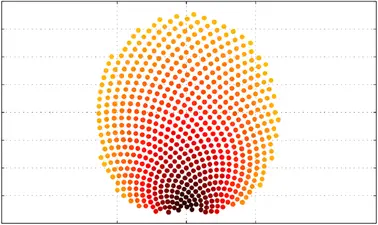There is 0 item now in your comparison listView a comparison list

# Mathematical Optimization for Engineers

Price
Free
3,193 people completed this program

## Overview

Today, for almost every product on the market and almost every service offered, some form of optimization has played a role in their design.

However, optimization is not a button-press technology. To apply it successfully, one needs expertise in formulating the problem, selecting and tuning the solution algorithm and finally, checking the results. We have designed this course to make you such an expert.

This course is useful to students of all engineering fields. The mathematical and computational concepts that you will learn here have application in machine learning, operations research, signal and image processing, control, robotics and design to name a few.

We will start with the standard unconstrained problems, linear problems and general nonlinear constrained problems. We will then move to more specialized topics including mixed-integer problems; global optimization for non-convex problems; optimal control problems; machine learning for optimization and optimization under uncertainty. Students will learn to implement and solve optimization problems in Python through the practical exercises.

• Mathematical definitions of objective function, degrees of freedom, constraints and optimal solution
• Mathematical as well as intuitive understanding of optimality conditions
• Different optimization formulations (unconstrained v/s constrained; linear v/s nonlinear; mixed-integer v/s continuous; time-continuous or dynamic; optimization under uncertainty)
• Fundamentals of the solution methods for each these formulations
• Optimization with machine learning embedded
• Hands-on training in implementing and solving optimization problems in Python, as exercises

## Syllabus

**Week 1:**Introduction and math review

• Mathematical definitions of objective function, degrees of freedom, constraints and optimal solution with real-world examples
• Review of some mathematical basics needed to take us through the course

**Week 2:**Unconstrained optimization

• Basics of iterative descent: step direction and step length
• Common algorithms like steepest descent, Newton’s method and its variants and trust-region methods.

**Week 3:**Linear optimization

• KKT conditions of optimality for constrained problems
• Simplex method
• Interior point methods

**Week 4:**Nonlinear optimization

• Penalty, log-barrier and SQP methods

Mixed-integer optimization

• Branch and bound method for mixed-integer linear problems

**Week 5:**Global optimization

• Branch and bound method for nonlinear non-convex problems
• Constructing relaxations
• Different formulations and their numerical performance
• Stochastic methods, genetic algorithm and derivative free methods

**Week 6:**Dynamic optimization

• Full discretization, single-shooting and multi-shooting methods
• Nonlinear model predictive control

**Week 7:**Machine learning for optimization

• Mechanistic, data-driven and hybrid modelling
• Basics of training machine learning models
• Optimization with machine learning embedded

**Week 8:**Optimization under uncertainty

• Parametric optimization
• Two stage stochastic problems
• Robust optimization via semi-infinite problems

## Authored by

RWTH Aachen University

## Reviews(0)

Help others make their choice. Be the first one to leave a review

Leave a reviewBeginner
Certification
English
8 weeks
Self-paced
Online